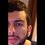# Physics fact for those who take photos of themselves in front of a mirror

Does your bathroom mirror show you older or younger than you actually are ? Compute an order of magnitude estimate for the age difference

The image formed of an object placed in front of a flat mirror is as far behind the mirror as the object is in front of it. A flat mirror produces an image that has an apparent front-back reversal

The image of the bathroom mirror shows you younger than you actually are

Let the distance between the observer and the mirror be 15 cm

s=15 cm = 0.15 m

the reflected image seen by the person is from the light that traveled from the person to the mirror and back. The time interval is calculated using the following formula:

t= (2s)/(c)

c= speed of light = 3.00 * 10^8

the reflected light gives the image of what the person had been earlier

t= (2 * 0.15)/(3.00 * 10^8) = 10^-9 seconds

Therefore, the person appeared as 10^-9 seconds younger than he actually isNote by Jaber Al-arbash
6 years, 9 months ago

This discussion board is a place to discuss our Daily Challenges and the math and science related to those challenges. Explanations are more than just a solution — they should explain the steps and thinking strategies that you used to obtain the solution. Comments should further the discussion of math and science.

When posting on Brilliant:

• Use the emojis to react to an explanation, whether you're congratulating a job well done , or just really confused .
• Ask specific questions about the challenge or the steps in somebody's explanation. Well-posed questions can add a lot to the discussion, but posting "I don't understand!" doesn't help anyone.
• Try to contribute something new to the discussion, whether it is an extension, generalization or other idea related to the challenge.

MarkdownAppears as
*italics* or _italics_ italics
**bold** or __bold__ bold
- bulleted- list
• bulleted
• list
1. numbered2. list
1. numbered
2. list
Note: you must add a full line of space before and after lists for them to show up correctly
paragraph 1paragraph 2

paragraph 1

paragraph 2

[example link](https://brilliant.org)example link
> This is a quote
This is a quote
    # I indented these lines
# 4 spaces, and now they show
# up as a code block.

print "hello world"
# I indented these lines
# 4 spaces, and now they show
# up as a code block.

print "hello world"
MathAppears as
Remember to wrap math in $$ ... $$ or $ ... $ to ensure proper formatting.
2 \times 3 $2 \times 3$
2^{34} $2^{34}$
a_{i-1} $a_{i-1}$
\frac{2}{3} $\frac{2}{3}$
\sqrt{2} $\sqrt{2}$
\sum_{i=1}^3 $\sum_{i=1}^3$
\sin \theta $\sin \theta$
\boxed{123} $\boxed{123}$

Sort by:

Wow! Never thought that...amazing

- 6 years, 9 months ago

wow..never thought of that thing

- 6 years, 9 months ago